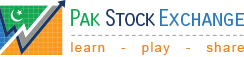Calculate Fair Value of a Pakistani Stock using Discounted Cash Flow### Fair Value Calculator

#### Discounted Cash Flow Method

Discounted cash flow (DCF) is a valuation method used to estimate the value of an investment based on its future cash flows. DCF analysis finds the present value of expected future cash flows using a discount rate. A present value estimate is then used to evaluate a potential investment. If the value calculated through DCF is higher than the current cost of the investment, the opportunity should be considered.

### Calculate

Enter company's Earning Per Share for the last 12 months or expected 12-month earnings per share. You can find earning per share information in the company's latest financial statements.

Rs.

Enter a percentage amount of earning growth rate that you expect the company will post for the next few years. This is one of the most important factors in calculating the fair value of any company. A value of 0 means no growth (or constant earnings) in the next few years. Very high growth companies may have 50% earnings increase per year.

%

Type number of years that you expect the growth entered in previous field will continue.

Enter a percentage amount of earning growth rate that you expect the company will post in long term. This is usually less than the initial years' growth rate, because companies grow rapidly in early years and then their earnings become stable. A value of 0 means you no growth is expected in the long term.

%

This factor is also very important in estimating the fair value. This rate depends upon many factors, including risk free rates, market conditions, volatility of the particular stock, debt/equity ratio of the company, and other risk related measures. Typical values are between 15 and 40. The more the risk, the higher this discount rate value.

%

### Random Financial Term

View More

#### Price-to-Book Ratio (P/B)

What you Need ?

Balance Sheet, Most Recent Stock Price

Formula

P/B Ratio = Price per Share / Book Value per Share

Meaning

Book value (BV) is already listed on the balance sheet, it's just under a different name: shareholder equity. Equity is the portion of the company that owners (i.e. shareholders) own free and clear. Dividing book value by the number of shares outstanding gives you book value per share.
Like P/E, the P/B ratio is essentially the number of dollars you'll have to pay for \$1 of equity. And like P/E, there are different criteria for what makes a P/B ratio "high" or "low."

### Top Players

Based on 2020-01-16 data
Player Profit
Jamal
Karachi, Pakistan
+17.7%
Mujeeb